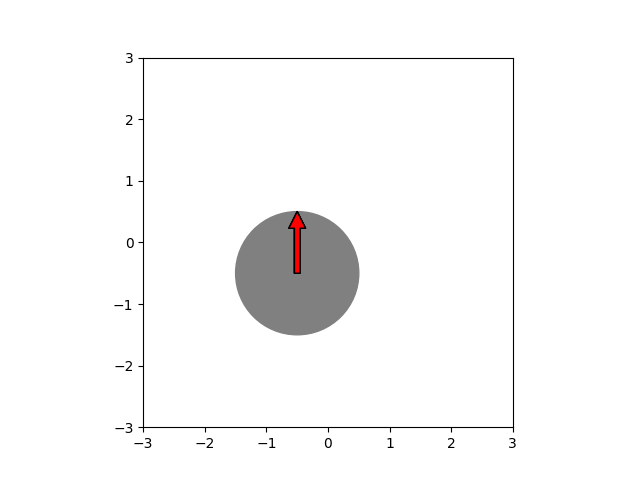# about animation of quiver vector arrows (mathematical vectors)

Hi there,

I am trying to find out if it is possible to add vectors based off of quiver in matplotlib and animate this via matplotlib animation.
I was able to use quiver to create static graphs of vector addition.

I am trying to duplicate the animation style here from this link:
https://www.physics.mun.ca/~jjerrett/vector/vector.html

But i saw on stackoverflow that at one point it was not possible to position vectors
then they said it can, see this link:

So to explain details of my program, it asks user to input 2 vectors.
Then the 2 vectors, the tails, are placed at the origin.
Then need to move one of the vector tails to the tip of the other vector in order to do vector addition.
And then draw arrow from origin to the final point.

Hope to get some help on this.

Paluee

1 Like

I am the author of that SO post you link to and have removed the first sentence because it was confusing (I was apologizing for having made an error, not saying that it could not be done at some point in the past).

If you only want to move around a few vectors, using `ax.quiver` is probably overkill in terms of complexity. I suggest instead you look at `ax.annotate` but with no text (which is a bit odd, but it works)

To demonstrate this I wrote a crude matplotclock:

``````import matplotlib.pyplot as plt
import numpy as np
import matplotlib.patches as mpatches
from matplotlib.animation import FuncAnimation

fig, ax = plt.subplots()
an1 = ax.annotate("", xy=(0, 1), xytext=(0, 0), arrowprops={"facecolor": "black"})
an2 = ax.annotate("", xy=(0, 1), xytext=(0, 0), arrowprops={"facecolor": "blue"})
an3 = ax.annotate("", xy=(0, 1), xytext=(0, 0), arrowprops={"facecolor": "red"})
face = mpatches.Circle((0, 0), radius=1, zorder=-1, color="gray")

ax.set_xlim(-3, 3)
ax.set_ylim(-3, 3)
ax.set_aspect("equal")

def anim(j):
# compute the new center
center = np.array(np.unravel_index(j % 100, (10, 10))) / 10 - 0.5
# set the clock face to that center
face.set_center(center)

# advance time 15 degree / frame
j *= 15
# compute the angles of the second, minute, and hour hands
h = np.deg2rad(j / (60 * 60))

# adjust the end of the annotation with the arrow on it
an1.xy = center + (np.sin(h), np.cos(h))
an2.xy = center + (np.sin(m), np.cos(m))
an3.xy = center + (np.sin(s), np.cos(s))

# adjust the end of the annotation without the arrow
an1.set_position(center)
an2.set_position(center)
an3.set_position(center)

# return the Artists so blitting works
return [an1, an2, an3, face]

anim = FuncAnimation(fig, anim, frames=60, blit=True)
plt.show()

``````For more details about how you can customize the annotation see https://matplotlib.org/3.1.1/tutorials/text/annotations.html#sphx-glr-tutorials-text-annotations-py and https://matplotlib.org/3.1.1/api/_as_gen/matplotlib.axes.Axes.annotate.html#matplotlib.axes.Axes.annotate

Hi,
I tried your code in JupyterLab, and initially it produced errors a few days ago. Then I just tried it again, it completed without any errors the first run, but did not produce anything. Then i tried to run the code a second time, and got the same error as before. It is a big long Trace of error stemming in the FuncAnimate function.
So not sure what exactly is the error.
P.

Can you post the exception? It is very hard to guess what the problem is (at it "works on my system ").

One guess is that you are using the `inline` backend. This example will not work with the inline backend (and animation in general will not work with the inline backend) because the figure is destroyed after the first time it is shown.

I suggest you install ipympl and use `%matplotlib widget`, run the example as a script, or run the script at the normal IPython prompt.

Hi Tacaswell, I am guessing its my environment (the ipython notebooks) like you said using 'inline backend" is probably the issue. I see a window generated in using an older version of jupyter, then it becomes a static picture but a bit blurred i guess an attempt at going into transition to another frame or something. I will take your advice and try it out.